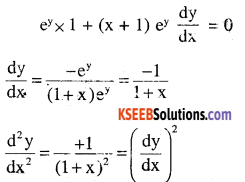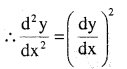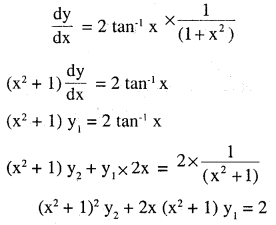# 2nd PUC Maths Question Bank Chapter 5 Continuity and Differentiability Ex 5.7

Students can Download Maths Chapter 5 Continuity and Differentiability Ex 5.7 Questions and Answers, Notes Pdf, 2nd PUC Maths Question Bank with Answers helps you to revise the complete Karnataka State Board Syllabus and score more marks in your examinations.

## Karnataka 2nd PUC Maths Question Bank Chapter 5 Continuity and Differentiability Ex 5.7

### 2nd PUC Maths Continuity and Differentiability NCERT Text Book Questions and Answers Ex 5.7

Find the second order derivatives of the functions given in Exercises 1 to 10.

Question 1.
x2 + 3x + 2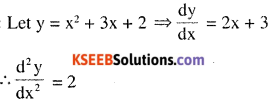Question 2.
x20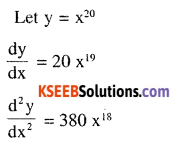Question 3.
x. cos x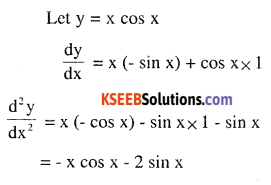Question 4.
log x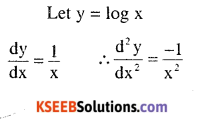Question 5.
x3 log x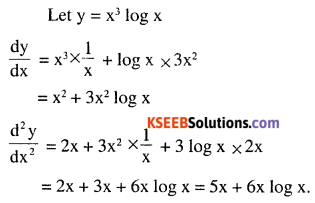Question 6.
ex sin 5x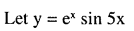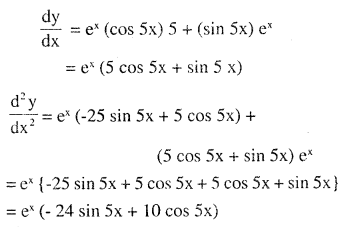Question 7.
e6x cos 3x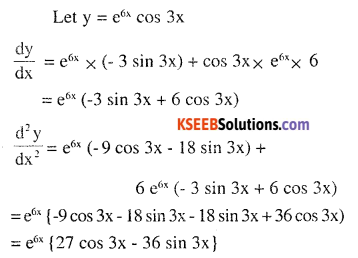Question 8.
tan-1 x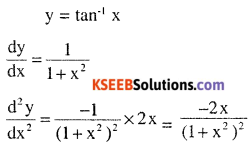Question 9.
log  (log x)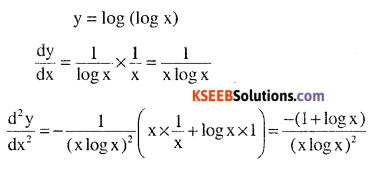Question 10.
sin(log x)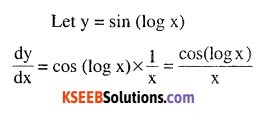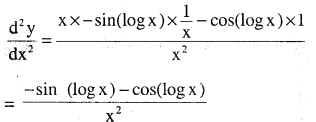Question 11.
If y = 5 cos x – 3 sin x prove that $$\frac{d^{2} y}{d x^{2}}+y=0$$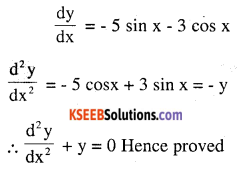Question 12.
$$\text { If } y=\cos ^{-1} x, \text { Find } \frac{d^{2} y}{d x^{2}}$$
in terns of y alone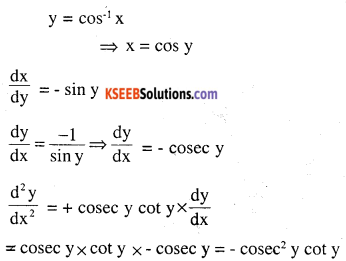Question 13.
If y = 3 cos (log x) + 4 sin (log x), show that x2y2 + xy1 + y = 0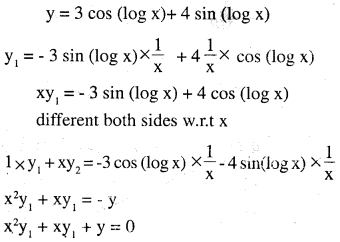Question 14.
If y = Ae-nx + Benx, Show that
$$\frac{d^{2} y}{d x^{2}}-(m+n) \frac{d y}{d x}+m n y=0$$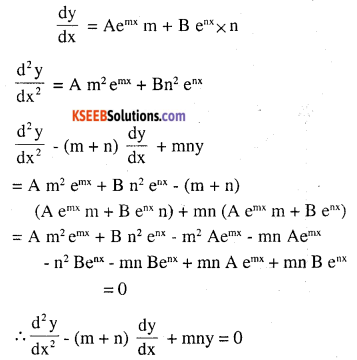Question 15.
If y = 500e7x + 600e-7x, show that $$\frac{d^{2} y}{d x^{2}}=49 y$$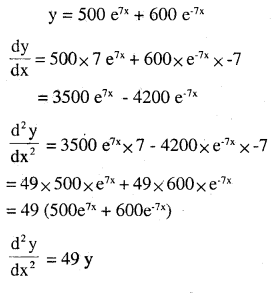Question 16.
$$\text { If } \mathrm{e}^{y}(x+1)=1, \text { show that } \frac{d^{2} y}{d x^{2}}=\left(\frac{d y}{d x}\right)^{2}$$